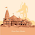## Saturday, January 29, 2011

### Problems and Solutions

IPhO 2009 (XL Merida, Mexico)
Theoretical Problem 1 (pdf)
Theoretical Problem 2 (pdf)
Theoretical Problem 3 (pdf)
Experimental Problem 1 (pdf)
Experimental Problem 2 (pdf)

IPhO 2008 (XXXIX Hanoi, Vietnam)
Theoretical Problem 1 (pdf)
Theoretical Problem 2 (pdf)
Theoretical Problem 3 (pdf)
Experimental Problem (pdf)
Theoretical Solution 1 (pdf)
Theoretical Solution 2 (pdf)
Theoretical Solution 3 (pdf)
Experimental Solution (pdf)

IPhO 2007 (XXXVIII Isfahan, Iran)
Theoretical Question (Orange) Problem (pdf)
Theoretical Question (Blue) Problem (pdf)
Theoretical Question (Pink) Problem (pdf)
Experimental Question (Green) Problem (pdf)
Theoretical Question (Orange) Solution (pdf)
Theoretical Question (Blue) Solution (pdf)
Theoretical Question (Pink) Solution (pdf)
Experimental Question (Green) Solution (pdf)
Experimental Question (Green) Description (pdf)

IPhO 2006 (XXXVII Singapore, Singapore)
Theoretical Question 1 Problem (pdf)
Theoretical Question 2 Problem (pdf)
Theoretical Question 3 Problem (pdf)
Experimental Question Problem (pdf)
Theoretical Question 1 Solution (pdf)
Theoretical Question 2 Solution (pdf)
Theoretical Question 3 Solution (pdf)
Experimental Question Solution (pdf)

IPhO 2005 (XXXVI Salamanca, Spain)

IPhO 2004 (XXXV Pohang, South Korea)
Theoretical Question 1 Problem (pdf)
Theoretical Question 2 Problem (pdf)
Theoretical Question 3 Problem (pdf)
Experimental Question Problem (pdf)
 Preview (Powerpoint) Answer Form (pdf) Theoretical Question 2 Solution (pdf) Preview (Powerpoint) Answer Form (pdf) Theoretical Question 3 Solution (pdf) Preview (Powerpoint) Answer Form (pdf) Experimental Question Solution (pdf) Preview (Powerpoint) Answer Form (pdf)

IPhO 2003 (XXXIV Taipei, Taiwan)

IPhO 2002 (XXXIII Nusa Dua, Indonesia)
Theoretical Question 1 Problem (pdf)
Theoretical Question 2 Problem (pdf)
Theoretical Question 3 Problem (pdf)
Experimental Question 1 Problem (pdf)
Experimental Question 2 Problem (pdf)
Theoretical Question 1 Solution (pdf)
Theoretical Question 2 Solution (pdf)
Theoretical Question 3 Solution (pdf)
Experimental Question 1 Solution (pdf)
Experimental Question 2 Solution (pdf)

IPhO 2001 (XXXII Antalya, Turkey)

 Theoretical Questions Problems (pdf) (post script format) (doc format) Experimental Questions Problems (pdf) (post script format) (doc format)

 Theoretical Questions Solutions (pdf) (post script format) (doc format) Experimental Questions Solutions (pdf) (post script format) (doc format)

IPhO 2000 (XXXI Leicester, Great Britain)
Theoretical Question 1 Problem (html)
Theoretical Question 2 Problem (html)
Theoretical Question 3 Problem (html)
Theoretical Question 1 Problem (html)
Theoretical Question 2 Problem (html)
(Recommended to copy pictures on your own files.)

 Theoretical Question 1 Solution (jpg's) Theoretical Question 2 Solution (jpg's) Theoretical Question 3 Solution (jpg's) Figure1      Figure6 Figure11 Figure13 Figure2      Figure7 Figure12 Figure14 Figure3      Figure8 Figure4      Figure9 Figure5      Figure10

 (post script) Theoretical Question 2 Problem (zipped doc) (post script) Theoretical Question 3 Problem (zipped doc) (post script) Experimental Question Problem (zipped doc) (post script)

 Theoretical Question 1 Solution (zipped doc) (post script) Theoretical Question 2 Solution (zipped doc) (post script) Theoretical Question 3 Solution (zipped doc) (post script) Experimental Question Solution (zipped doc) (post script) Comment on the experimental problem (zipped doc) (post script)

IPhO 1998 (XXIX Reykjavik, Iceland)
The original english problems and solutions (zipped post script)
The Icelandic problems and solutions (zipped post script)

Problems and Solutions (pdf)

IPhO 1996 (XXVII Oslo, Norway)
Theoretical Problems and Solutions (pdf)
Experimental Problems and Solutions (pdf)

IPhO 1995 (XXVI Canberra, Australia)
Theoretical Questions Problems (pdf)
Experimental Question 1 Problem (pdf)
Experimental Question 2 Problem (pdf)
Theoretical Questions Solutions (pdf)
Experimental Question 1 Solution (pdf)
Experimental Question 2 Solution (pdf)

IPhO 1994 (XXV Beijing, China)
Problems and Solutions (doc)

IPhO 1993 (XXIV Williamsburg, USA)
Problems and Solutions (pdf)

IPhO 1992 (XXIII Helsinki-Espoo, Finland)
Theoretical Question 1 Problem and Solution (pdf)
Theoretical Question 2 Problem and Solution (pdf)
Theoretical Question 3 Problem and Solution (pdf)
Experimental Question 1 Problem and Solution (pdf)
Experimental Question 2 Problem and Solution (pdf)
Distribution of results (pdf)

IPhO 1991 (XXII Havana, Cuba)
Problems and Solutions (pdf)

IPhO 1990 (XXI Groningen, The Netherlands)
Problems and Solutions (pdf)

IPhO 1989 (XX Warsaw, Poland)
Problems and Solutions (doc)

IPhO 1988 (XIX Bad Ischl, Austria)
Problems and Solutions (pdf)

IPhO 1987 (XVIII Jena, GDR)
Problems and Solutions (pdf)

IPhO 1986 (XVII London-Harrow, Great Britain)
Theoretical Questions Problems (doc)
Experimental Questions Problems (doc)
Theoretical Questions Solutions (doc)
Experimental Questions Solutions (doc)

IPhO 1985 (XVI Portoroz, SFR Yugoslavia)
Problems and Solutions (pdf)

IPhO 1984 (XV Sigtuna, Sweden)
Problems and Solutions (doc)

IPhO 1983 (XIV Bucharest, Romania)
Theoretical Ouestions (doc)
Solution and marking scheme for theoretical question I (Mechanics).
Solution and marking scheme for theoretical question II (Electricity).
Solution and marking scheme for theoretical question III (Optics).
Solution and marking scheme for theoretical question IV (Atomics).
Solution for IPhO's logo question.

IPhO 1982 (XIII Malente, FRG)
Problems and Solutions (pdf)

IPhO 1981 (XII Varna, Bulgaria)
Theoretical Problems and Solutions
Experimental Problems and Solutions

There were no IPhO in 1980

IPhO 1979 (XI Moscow, Soviet Union)
Problems and Solutions (doc)

There were no IPhO in 1978

IPhO 1977 (X Hradec Kralove, Czechoslovakia)
Problems and Solutions (pdf)

IPhO 1976 (IX Budapest, Hungary)
(The document contains also IPhO 1968)

Problems and Solutions (doc)

IPhO 1975 (VIII Guestrow, GDR)
Problems and Solutions (pdf)

IPhO 1974 (VII Warsaw, Poland)
Problems and Solutions (doc)

There were no IPhO in 1973

IPhO 1972 (VI Bucharest, Romania)
Problems and Solutions (doc)

IPhO 1971 (V Sofia, Bulgaria)
Problems (doc)
Solutions (doc)

IPhO 1970 (IV Moscow, Soviet Union)
Problems and Solutions (doc)

IPhO 1969 (III Brno, Czechoslovakia)
(Only theoretical part)

Theoretical Problems (pdf)

IPhO 1968 (II Budapest, Hungary)
(The document contains also IPhO 1976)

Problems and Solutions (doc)

IPhO 1967 (I Warsaw, Poland)
Problems and Solutions (doc)

1.At this moment I am going away to do my breakfast, after having
my breakfast coming yet again to read more news.

Feel free to visit my homepage GFI Norte
Also see my website > GFI Norte

2.FABULOUS COLLECTION

3.Good collection

4.